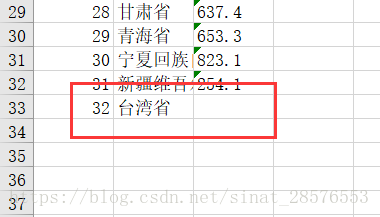﻿ 使用Python读取和修改Excel文件(基于xlrd、xlwt和openpyxl模块)_python_脚本之家
python# 使用Python读取和修改Excel文件(基于xlrd、xlwt和openpyxl模块)

## 1、使用xlrd模块对xls文件进行读操作### 1.1 获取工作簿对象

```
import xlrd  #引入模块

#打开文件，获取excel文件的workbook（工作簿）对象
workbook=xlrd.open_workbook("DataSource/Economics.xls")  #文件路径

```

### 1.2 获取工作表对象

```'''对workbook对象进行操作'''

#获取所有sheet的名字
names=workbook.sheet_names()
print(names) #['各省市', '测试表']  输出所有的表名，以列表的形式

#通过sheet索引获得sheet对象
worksheet=workbook.sheet_by_index(0)
print(worksheet)  #<xlrd.sheet.Sheet object at 0x000001B98D99CFD0>

#通过sheet名获得sheet对象
worksheet=workbook.sheet_by_name("各省市")
print(worksheet) #<xlrd.sheet.Sheet object at 0x000001B98D99CFD0>

#由上可知，workbook.sheet_names() 返回一个list对象，可以对这个list对象进行操作
sheet0_name=workbook.sheet_names()  #通过sheet索引获取sheet名称
print(sheet0_name)  #各省市
```

### 1.3 获取工作表的基本信息

```'''对sheet对象进行操作'''
name=worksheet.name  #获取表的姓名
print(name) #各省市

nrows=worksheet.nrows  #获取该表总行数
print(nrows)  #32

ncols=worksheet.ncols  #获取该表总列数
print(ncols) #13```

### 1.4 按行或列方式获得工作表的数据

```for i in range(nrows): #循环打印每一行
print(worksheet.row_values(i))  #以列表形式读出，列表中的每一项是str类型
#['各省市', '工资性收入', '家庭经营纯收入', '财产性收入', ………………]
#['北京市', '5047.4', '1957.1', '678.8', '592.2', '1879.0，…………]

col_data=worksheet.col_values(0)  #获取第一列的内容
print(col_data)
```

1.5 获取某一个单元格的数据

我们还可以将查询精确地定位到某一个单元格。

```#通过坐标读取表格中的数据
cell_value1=sheet0.cell_value(0,0)
cell_value2=sheet0.cell_value(1,0)
print(cell_value1)  #各省市
print(cell_value2)   #北京市

cell_value1=sheet0.cell(0,0).value
print(cell_value1) #各省市
cell_value1=sheet0.row(0).value
print(cell_value1)  #各省市```

## 2、使用xlwt模块对xls文件进行写操作

### 2.1 创建工作簿

```
# 导入xlwt模块
import xlwt

#创建一个Workbook对象，相当于创建了一个Excel文件
book=xlwt.Workbook(encoding="utf-8",style_compression=0)

'''
Workbook类初始化时有encoding和style_compression参数
encoding:设置字符编码，一般要这样设置：w = Workbook(encoding='utf-8')，就可以在excel中输出中文了。默认是ascii。
style_compression:表示是否压缩，不常用。
'''```

### 2.2 创建工作表

```
# 创建一个sheet对象，一个sheet对象对应Excel文件中的一张表格。
# 其中的test是这张表的名字,cell_overwrite_ok，表示是否可以覆盖单元格，其实是Worksheet实例化的一个参数，默认值是False```

### 2.3 按单元格的方式向工作表中添加数据

```
# 向表test中添加数据
sheet.write(0, 0, '各省市')  # 其中的'0-行, 0-列'指定表中的单元，'各省市'是向该单元写入的内容
sheet.write(0, 1, '工资性收入')

#也可以这样添加数据
txt1 = '北京市'
sheet.write(1,0, txt1)
txt2 = 5047.4
sheet.write(1, 1, txt2)```

最后被文件被保存之后，上文语句形成的“工作表”如下所示:### 2.4 按行或列方式向工作表中添加数据

```
#添加第二个表

Province=['北京市', '天津市', '河北省', '山西省', '内蒙古自治区', '辽宁省',
'吉林省', '黑龙江省', '上海市', '江苏省', '浙江省', '安徽省', '福建省',
'江西省', '山东省', '河南省', '湖北省', '湖南省', '广东省', '广西壮族自治区',
'海南省', '重庆市', '四川省', '贵州省', '云南省', '西藏自治区', '陕西省', '甘肃省',
'青海省', '宁夏回族自治区', '新疆维吾尔自治区']

Income=['5047.4', '3247.9', '1514.7', '1374.3', '590.7', '1499.5', '605.1', '654.9',
'6686.0', '3104.8', '3575.1', '1184.1', '1855.5', '1441.3', '1671.5', '1022.7',
'1199.2', '1449.6', '2906.2', '972.3', '555.7', '1309.9', '1219.5', '715.5', '441.8',
'568.4', '848.3', '637.4', '653.3', '823.1', '254.1']

Project=['各省市', '工资性收入', '家庭经营纯收入', '财产性收入', '转移性收入', '食品', '衣着',
'居住', '家庭设备及服务', '交通和通讯', '文教、娱乐用品及服务', '医疗保健', '其他商品及服务']

#填入第一列
for i in range(0, len(Province)):
sheet2.write(i+1, 0, Province[i])

#填入第二列
for i in range(0,len(Income)):
sheet2.write(i+1,1,Income[i])

#填入第一行
for i in range(0,len(Project)):
sheet2.write(0,i,Project[i])```

### 2.5 保存创建的文件

```# 最后，将以上操作保存到指定的Excel文件中
book.save('DataSource\\test1.xls')  ```## 3、使用openpyxl模块对xlsx文件进行读操作

### 3.1 获取工作簿对象

```import openpyxl

#获取 工作簿对象
#与xlrd 模块的区别
#wokrbook=xlrd.open_workbook(""DataSource\Economics.xls)```

### 3.2 获取所有工作表名

```#获取工作簿 workbook的所有工作表
shenames=workbook.get_sheet_names()
print(shenames)  #['各省市', '测试表']
#在xlrd模块中为 sheetnames=workbook.sheet_names()

#使用上述语句会发出警告：DeprecationWarning: Call to deprecated function get_sheet_names (Use wb.sheetnames).
#说明 get_sheet_names已经被弃用 可以改用 wb.sheetnames 方法
shenames=workbook.sheetnames
print(shenames)  #['各省市', '测试表']```

### 3.3 获取工作表对象

```#获得工作簿的表名后，就可以获得表对象
worksheet=workbook.get_sheet_by_name("各省市")
print(worksheet)  #<Worksheet "各省市">

#使用上述语句同样弹出警告：DeprecationWarning: Call to deprecated function get_sheet_by_name (Use wb[sheetname]).
#改写成如下格式
worksheet=workbook["各省市"]
print(worksheet)  #<Worksheet "各省市">

#还可以通过如下写法获得表对象
worksheet1=workbook[shenames]
print(worksheet1)  #<Worksheet "测试表">```

3.4 根据索引方式获取工作表对象

```#还可以通过索引方式获取表对象
worksheet=workbook.worksheets
print(worksheet) #<Worksheet "各省市">

#也可以用如下方式
#获取当前活跃的worksheet,默认就是第一个worksheet
ws = workbook.active```

### 3.5 获取工作表的属性

```#经过上述操作，我们已经获得了第一个“表”的“表对象“，接下来可以对表对象进行操作

name=worksheet.title  #获取表名
print(name)  #各省市
#在xlrd中为worksheet.name

#获取该表相应的行数和列数
rows=worksheet.max_row
columns=worksheet.max_column
print(rows,columns)  #32 13
#在xlrd中为 worksheet.nrows  worksheet.ncols```

### 3.6 按行或列方式获取表中的数据

sheet.rows，这是一个生成器，里面是每一行数据，每一行数据由一个元组类型包裹。

sheet.columns，同上，里面是每一列数据。

```for row in worksheet.rows:
for cell in row:
print(cell.value,end=" ")
print()
"""

……
"""

for col in worksheet.columns:
for cell in col:
print(cell.value,end=" ")
print()

'''

……
'''```

```
for row in worksheet.rows:
print(row)
'''
(<Cell '各省市'.A1>, <Cell '各省市'.B1>, <Cell '各省市'.C1>, <Cell '各省市'.D1>, <Cell '各省市'.E1>,……
(<Cell '各省市'.A2>, <Cell '各省市'.B2>, <Cell '各省市'.C2>, <Cell '各省市'.D2>, <Cell '各省市'.E2>, ……
……
'''
#可知，需要二次迭代

for row in worksheet.rows:
for cell in row:
print(cell,end=" ")
print()

'''
<Cell '各省市'.A1> <Cell '各省市'.B1> <Cell '各省市'.C1> <Cell '各省市'.D1>……
<Cell '各省市'.A2> <Cell '各省市'.B2> <Cell '各省市'.C2> <Cell '各省市'.D2> ……
……
'''
#还需要cell.value
for row in worksheet.rows:
for cell in row:
print(cell.value,end=" ")
print()```

### 3.7 获取特定行或特定列的数据

```#输出特定的行
for cell in list(worksheet.rows):  #获取第四行的数据
print(cell.value,end=" ")
print()
#河北省 1514.7 2039.6 107.7 139.8 915.5 167.9 531.7 115.8 285.7 265.4 166.3 47.0

#输出特定的列
for cell in list(worksheet.columns):  #获取第三列的数据
print(cell.value,end=" ")
print()
#家庭经营纯收入 1957.1 2707.4 2039.6 1622.9 2406.2 2210.8 2556.7 2521.5 767.7 2271.4 3084.3……

#已经转换成list类型，自然是从0开始计数。```

### 3.8 获取某一块的数据

```for rows in list(worksheet.rows)[0:3]:
for cell in rows[0:3]:
print(cell.value,end=" ")
print()
'''

'''

for i in range(1, 4):
for j in range(1, 4):
print(worksheet.cell(row=i, column=j).value,end=" ")
print()
'''

'''```

### 3.9 获取某一单元格的数据

```#精确读取表格中的某一单元格
content_A1= worksheet['A1'].value
print(content_A1)

content_A1=worksheet.cell(row=1,column=1).value
#等同于 content_A1=worksheet.cell(1,1).value
print(content_A1)
#此处的行数和列数都是从1开始计数的，而在xlrd中是由0开始计数的```

## 4、使用openpyxl模块对xlsx文件进行写操作

### 4.1 创建工作簿和获取工作表

```import openpyxl

# 创建一个Workbook对象，相当于创建了一个Excel文件
workbook=openpyxl.Workbook()
#wb=openpyxl.Workbook(encoding='UTF-8')

#获取当前活跃的worksheet,默认就是第一个worksheet
worksheet = workbook.active
worksheet.title="mysheet"```

4.2 创建新的工作表

```worksheet2 = workbook.create_sheet()   #默认插在工作簿末尾
#worksheet2 = workbook.create_sheet(0)  #插入在工作簿的第一个位置
worksheet2.title = "New Title"```

### 4.3 将数据写入工作表

```#以下是我们要写入的数据
Province=['北京市', '天津市', '河北省', '山西省', '内蒙古自治区', '辽宁省',
'吉林省', '黑龙江省', '上海市', '江苏省', '浙江省', '安徽省', '福建省',
'江西省', '山东省', '河南省', '湖北省', '湖南省', '广东省', '广西壮族自治区',
'海南省', '重庆市', '四川省', '贵州省', '云南省', '西藏自治区', '陕西省', '甘肃省',
'青海省', '宁夏回族自治区', '新疆维吾尔自治区']

Income=['5047.4', '3247.9', '1514.7', '1374.3', '590.7', '1499.5', '605.1', '654.9',
'6686.0', '3104.8', '3575.1', '1184.1', '1855.5', '1441.3', '1671.5', '1022.7',
'1199.2', '1449.6', '2906.2', '972.3', '555.7', '1309.9', '1219.5', '715.5', '441.8',
'568.4', '848.3', '637.4', '653.3', '823.1', '254.1']

Project=['各省市', '工资性收入', '家庭经营纯收入', '财产性收入', '转移性收入', '食品', '衣着',
'居住', '家庭设备及服务', '交通和通讯', '文教、娱乐用品及服务', '医疗保健', '其他商品及服务']

#写入第一行数据，行号和列号都从1开始计数
for i in range(len(Project)):
worksheet.cell(1, i+1,Project[i])

#写入第一列数据，因为第一行已经有数据了，i+2
for i in range(len(Province)):
worksheet.cell(i+2,1,Province[i])

#写入第二列数据
for i in range(len(Income)):
worksheet.cell(i+2,2,Income[i])```

### 4.4 保存工作簿

`workbook.save(filename='DataSource\\myfile.xlsx')`## 5、修改已经存在的工作簿(表)

### 5.1 插入一列数据

```import openpyxl

worksheet=workbook.worksheets

#在第一列之前插入一列
worksheet.insert_cols(1)  #

for index,row in enumerate(worksheet.rows):
if index==0:
row.value="编号"  #每一行的一个row就是第一列
else:
row.value=index
#枚举出来是tuple类型，从0开始计数

workbook.save(filename="DataSource\myfile.xlsx")```### 5.2 修改特定单元格

```worksheet.cell(2,3,'0')
worksheet["B2"]="Peking"```### 5.3 批量修改数据

```taiwan=[32,"台湾省"]
worksheet.append(taiwan)```